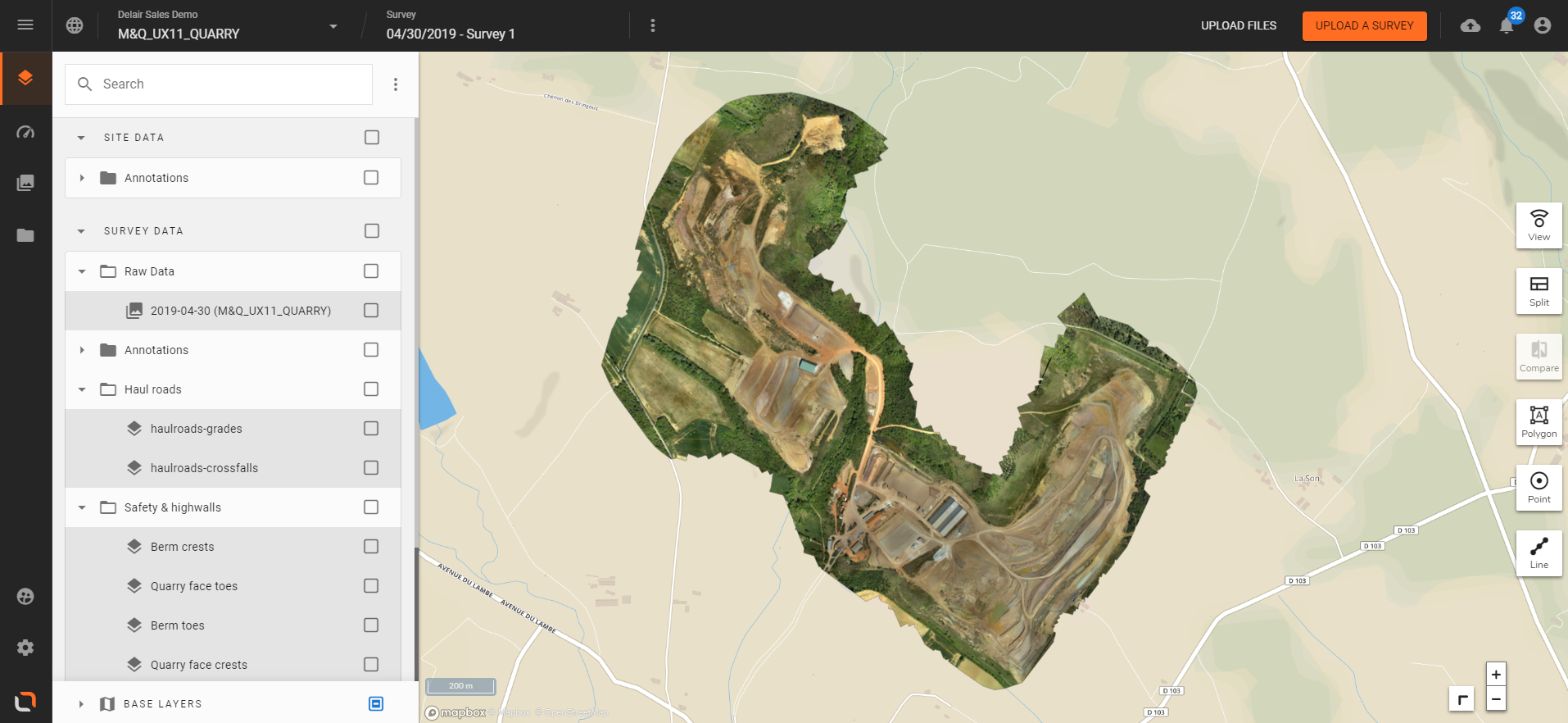# Volume Calculations

In this tutorial we review how to calculate a volume from a DSM (Digital Surface Model) in delair.ai. Then we present the different calculation methods currently available in the platform.

## 1. Direct calculation from a polygon annotation

Step 1 - Open your Site and select the adequate Survey in delair.ai on a 2D view. In example below we display the orthomap from the Base Layers.Step 2 - Zoom in your area of interest and draw a polygon annotation onto your 2D layer (orthomap, DSM) to determine the contour (base) from which volume will be calculated; using elevation data contained in DSM; in all polygon inner area. You may use DSM or Contour Lines in order to help you delimitate the calculation zone :

 ORTHOMOSAIC DSMDSM + CONTOUR LINESStep 3 - In this example we draw a simple polygon to get an immediate estimation of the volume of a bulk material pile :Step 4 - Click on polygon to open information panel on right side, then go to Volume Calculation section :Depending on the method selected (and that can be changed from the drop-down menu), an instant result will be displayed. For some methods, inputs from user are required.## 2. Exporting Volumes

In annotation information panel, below the volume figure, Press Save volume as vector in order to export results in geojson format :## 3. Available Methods

You can choose among six volume calculation methods :

 LOWEST POINTCalculates the volume between the surface model and a flat plane defined by the lowest point in the polygon
 TRIANGULATED SURFACECalculates the volume between the surface model and an interpolated base surface based on the elevation of points on the polygon boundary
 BEST FIT PLANEFits a flat plane minimizing the distance from the selected points to the plane
 CONFIGURABLE Z VALUECalculates the volume between the surface model and the selected polygon based on a flat-plane offset
 CONSTANT THICKNESSCalculates the volume between two flat planes based on entered elevations
 DIFFERENTIALCalculates the volume between the surface model and another survey surface or site surface